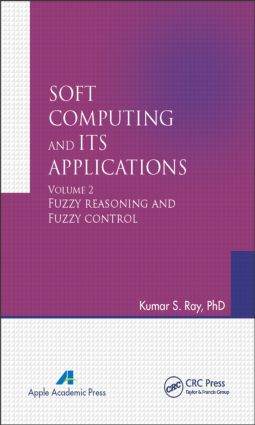# Soft Computing and Its Applications, Volume Two

## Fuzzy Reasoning and Fuzzy Control, 1st Edition

468 pages | 169 Color Illus.

Hardback: 9781771880466
pub: 2014-10-07
SAVE ~\$44.00
\$220.00
\$176.00
x
eBook (VitalSource) : 9780429095122
pub: 2014-10-07
from \$28.98

FREE Standard Shipping!

### Description

This is volume 2 of the two-volume Soft Computing and Its Applications. This volume discusses several advanced features of soft computing and hybrid methodologies. This new book essentially contains the advanced features of soft computing and different hybrid methodologies for soft computing. The book contains an abundance of examples and detailed design studies.

The tool soft computing can be a landmark paradigm of computation with cognition that directly or indirectly tries to replicate the rationality of human beings. The book explains several advanced features of soft computing, such as cognitive maps, complex valued fuzzy sets and fuzzy logic, quantum fuzzy sets and quantum fuzzy logic, and rough sets and hybrid methods that combine neural net fuzzy logic and genetic algorithms. The book contains several real-life applications to present the utility and potential of soft computing.

The book:

• Discusses the present state of art of soft computing

• Includes the existing application areas of soft computing

• Presents original research contributions

• Discusses the future scope of work in soft computing

The book is unique in that it bridges the gap between theory and practice, and it presents several experimental results on synthetic data and real-life data. The book provides a unified platform for applied scientists and engineers in different fields and industries for the application of soft computing tools in many diverse domains of engineering. This book can be used as a textbook and/or reference book by undergraduate and postgraduate students of many different engineering branches, such as electrical engineering, control engineering, electronics and communication engineering, computer sciences, and information sciences.

### Reviews

"This two-volume textbook set is a quite elementary, but rather comprehensive, introduction to the field of soft computing, accessible not only for undergraduates in mathematics, but also for students in computer science and engineering. The presentation is essentially correct, offers figures for most of the notions it defines, and presents lots of detailed numerical examples. Volume 1 starts with an explanation of the notion of soft computing and continues with chapters on fuzzy sets, fuzzy operators, fuzzy relations, fuzzy logic, fuzzy implications, fuzzy if-then models, and rough sets. Volume 2 covers in separate chapters the topics of fuzzy reasoning, fuzzy reasoning based on the concept of similarity, and fuzzy control."

—Siegfried J. Gottwald, writing in Zentralblatt MATH, 1308

Fuzzy Reasoning

Introduction

Model of approximate reasoning

Basic approach to Zadeh’s fuzzy reasoning

Extended fuzzy reasoning

Further extension of fuzzy reasoning

Generalized form of fuzzy reasoning

Application of fuzzy reasoning for prediction of radiation fog

Aggregation in fuzzy system modeling

Single Input Rule Modules (SIRMs) connected fuzzy reasoning method

Some properties of compositional rule of inference

Computation of compositional rule of inference under t-norms

Inverse approximate reasoning

Interpolative fuzzy reasoning

On generalized method-of-case inference rule

Generalized disjunctive syllogism

Ray’s bottom-up inferences

Multidimensional fuzzy reasoning based on multidimensional fuzzy implication

Fuzzy Reasoning Based on Concept of Similarity

Introduction

Fuzzy reasoning using similarity

Similarity based fuzzy reasoning method

Rule reduction is SBR

Proposed similarity measure

Fuzzy reasoning using similarity measures and computational rule of inference

Applications to different models

Reasoning based on total fuzzy similarity

Similarity-based bidirectional approximate reasoning

Logical approaches to fuzzy similarity-based reasoning

Fuzzy resolution based on similarity-based unification

Fuzzy Control

Introduction

Fuzzy controller

Illustration on basic approaches to fuzzy control

Fuzzy associative memory

Fuzzy controller design

Self-tuning of fuzzy controller

Single input rule module (SIRM)

Construction of PID controller by simplified fuzzy reasoning method

Fuzzy control as a fuzzy deduction system

Concluding Remarks

Review of the applications and future scope

Index